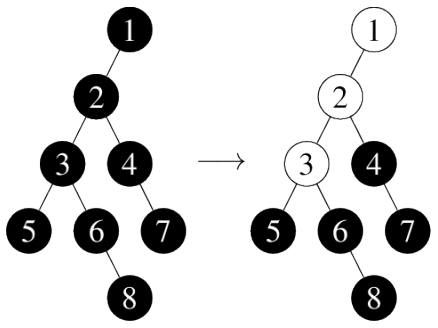시간 제한메모리 제한제출정답맞힌 사람정답 비율
1 초 128 MB128660.000%

## 문제

We have a “ball machine” that can be visualized as a rooted tree. The nodes of the tree are numbered from 1 to N. Each node is either empty or contains one ball. Initially, all nodes are empty. While running, the machine can perform operations of two different types:

1. Add k balls to the ball machine: Balls are put one by one into the root node. As long as a ball has an empty node directly beneath it, it will roll down. If there are multiple empty child nodes, the ball will choose the one which has the node with the smallest number in its subtree. So if the ball rolls down multiple levels, it makes a choice at each level. For example: If we add two balls to the machine in the picture below, they will go to nodes 1 and 3: The first ball rolls from node 4 to node 3 because node 3 is empty and it contains node 1 in its subtree (which consists of nodes 3 and 1); it further rolls from node 3 to node 1. The second ball rolls from node 4 to node 3 as well and stops there.1. Remove a ball from a specified node: This node becomes empty and balls from above (if there are any) roll down. Whenever a parent of an empty node contains a ball, this ball will roll down. If we remove balls from nodes 5, 7 and 8 (in this order) from the machine in the picture below, nodes 1, 2 and 3 will become empty.## 입력

The first line contains two integers N and Q – the number of tree nodes and the number of operations. The next N lines describe the ball machine. Each of these lines contains one integer, the number of a node: the i-th of these lines contains the number of node i’s parent node, or 0 if node i is the tree root. Each of the next Q lines contains two integers and describes an operation to be performed. An operation of type 1 is denoted by 1 k where k is the number of balls to be added to the machine. An operation of type 2 is denoted by 2 x where x is the number of the node from which a ball is to be removed. It is guaranteed that all performed operations are correct: Operations will not require to add more balls than there are empty nodes in the ball machine or to remove a ball from an empty node.

## 출력

For each operation of type 1, output the number of the node where the last inserted ball ended up. For each operation of type 2 output the number of balls that rolled down after removing the ball from the specified node.

## 제한

• N, Q ≤ 100 000.

## 예제 입력 1

8 4
0
1
2
2
3
3
4
6
1 8
2 5
2 7
2 8


## 예제 출력 1

1
3
2
2